• 遥感图像频率域增强,详细介绍几种图像频率域增强的方法
• 本文档包含数字图像处理(冈萨雷斯第三版)中的图像频率域滤波（傅里叶变换、巴特沃斯低通滤波高通滤波等）实验，包含整个实验过程和原理解释，以及详细的执行代码。代码复制后可在matlab中直接运行。
• 点击上方↑↑↑“OpenCV学堂”关注我代码演示如何在图像频率域实现卷积模糊与梯度提取图像频率域图像处理不仅可以在空间域进行还可以在频率域进行，把空间域的图像开窗卷积形式，变换得到频率域的矩阵点乘形式得到...


点击上方↑↑↑“OpenCV学堂”关注我代码演示如何在图像频率域实现卷积模糊与梯度提取图像频率域图像处理不仅可以在空间域进行还可以在频率域进行，把空间域的图像开窗卷积形式，变换得到频率域的矩阵点乘形式得到比较好的效果。转换到频率域最常见的是通过傅里叶变换得到图像的频率域表示，处理之后再反变换回去。支持各种卷积处理的效果，比如模糊，梯度提取等，OpenCV中支持傅里叶变换与逆变换的函数分别为# 傅里叶变换函数void cv::dft(    InputArray src,    OutputArray dst,    int flags = 0,    int nonzeroRows = 0 )# 傅里叶逆变换函数void cv::idft(    InputArray src,    OutputArray dst,    int flags = 0,    int nonzeroRows = 0 )低通滤波低通滤波可以看成是卷积模糊在频率域的表现形式，代码实现如下：def low_pass_filter_demo():    image = cv.imread("D:/images/test1.png", cv.IMREAD_GRAYSCALE)    img_float32 = np.float32(image)    rows, cols = image.shape    crow, ccol = rows//2 , cols//2    # FFT变换    dft = cv.dft(img_float32, flags = cv.DFT_COMPLEX_OUTPUT)    dft_shift = np.fft.fftshift(dft)    # 创建低通滤波器，低频区域为 1， 高频区域为 0    mask = np.zeros((rows, cols, 2), np.uint8)    mask[crow-30:crow+30, ccol-30:ccol+30] = 1    # 滤波    fshift = dft_shift*mask    # 逆变换    f_ishift = np.fft.ifftshift(fshift)    img_back = cv.idft(f_ishift)    img_back = cv.magnitude(img_back[:,:,0],img_back[:,:,1])    cv.normalize(img_back, img_back, 0, 1.0, cv.NORM_MINMAX)    cv.imshow("input", image);    cv.imshow("low-pass-filter", img_back)    cv.imwrite("D:/low_pass.png", np.uint8(img_back*255))    cv.waitKey(0)    cv.destroyAllWindows()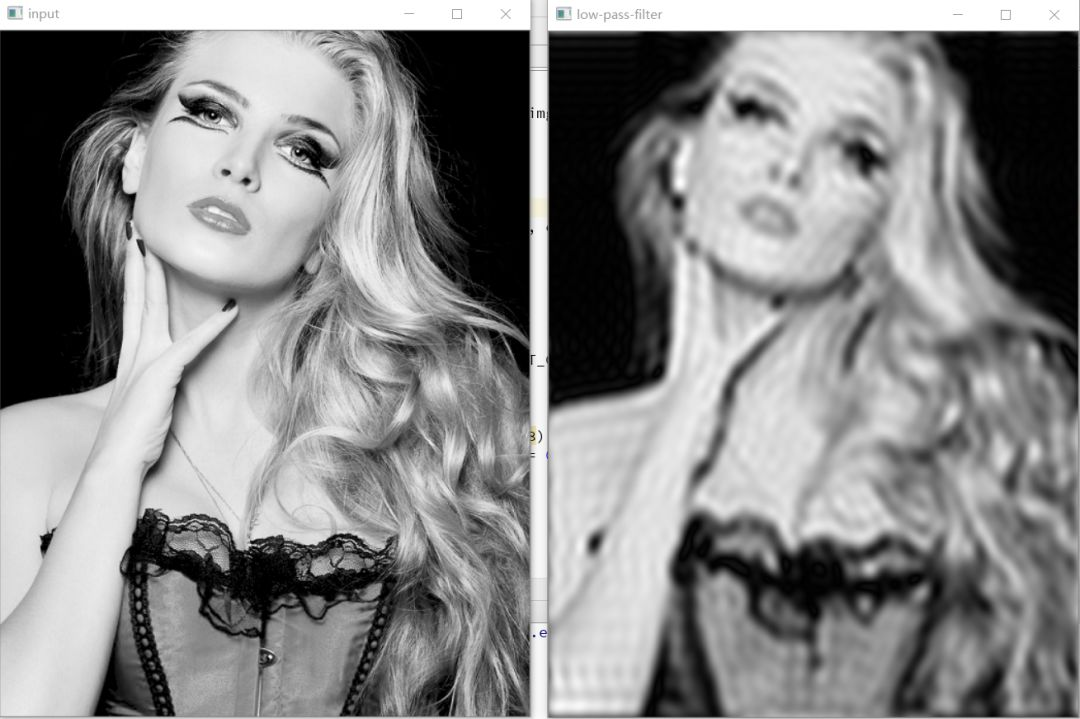高通滤波高通滤波可以看成是图像梯度在频率域的计算，代码实现如下：def high_pass_filter_demo():    image = cv.imread("D:/images/test1.png", cv.IMREAD_GRAYSCALE)    img_float32 = np.float32(image)    rows, cols = image.shape    crow, ccol = rows//2 , cols//2    # FFT变换    dft = cv.dft(img_float32, flags = cv.DFT_COMPLEX_OUTPUT)    dft_shift = np.fft.fftshift(dft)    # 创建高通滤波器，低频区域为 0， 高频区域为 1    mask = np.ones((rows, cols, 2), np.uint8)    mask[crow-30:crow+30, ccol-30:ccol+30] = 0    # 滤波    fshift = dft_shift*mask    # 逆变换    f_ishift = np.fft.ifftshift(fshift)    img_back = cv.idft(f_ishift)    img_back = cv.magnitude(img_back[:,:,0],img_back[:,:,1])    cv.normalize(img_back, img_back, 0, 1.0, cv.NORM_MINMAX)    cv.imshow("input", image);    cv.imshow("high-pass-filter", img_back)    cv.imwrite("D:/high_pass.png", np.uint8(img_back*255))    cv.waitKey(0)    cv.destroyAllWindows()运行效果如下：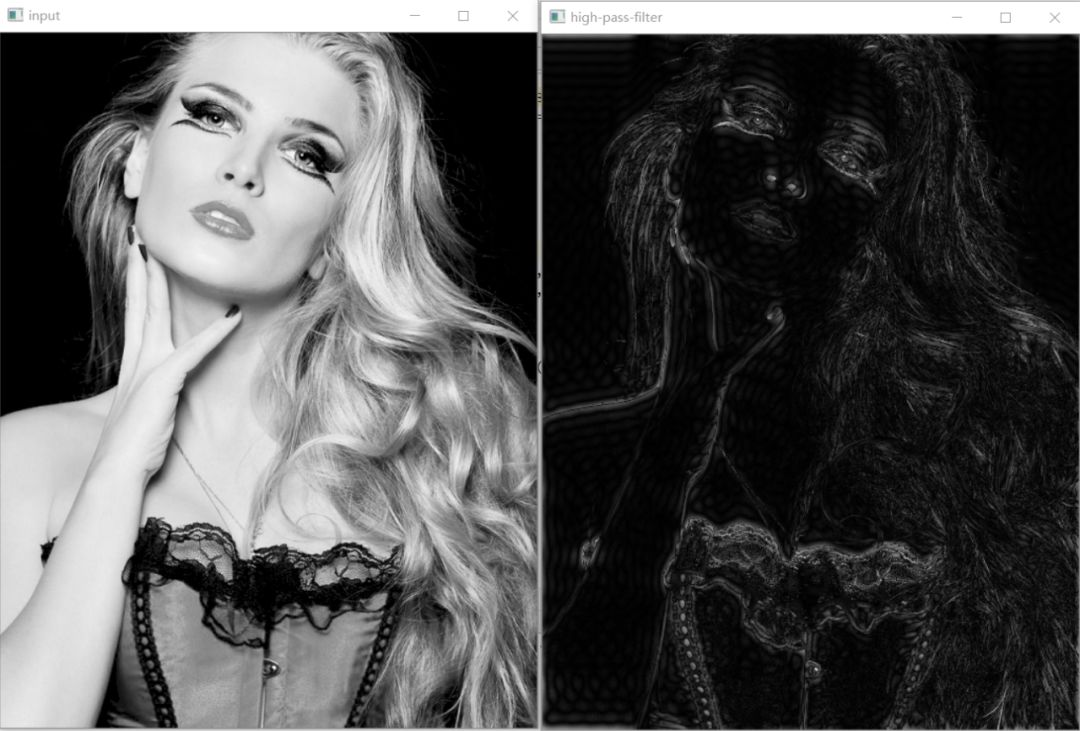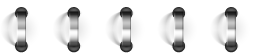往期精选告诉大家你 在看你需要知道的10种行人属性从零开始行人重识别OpenCV中如何读取URL图像文件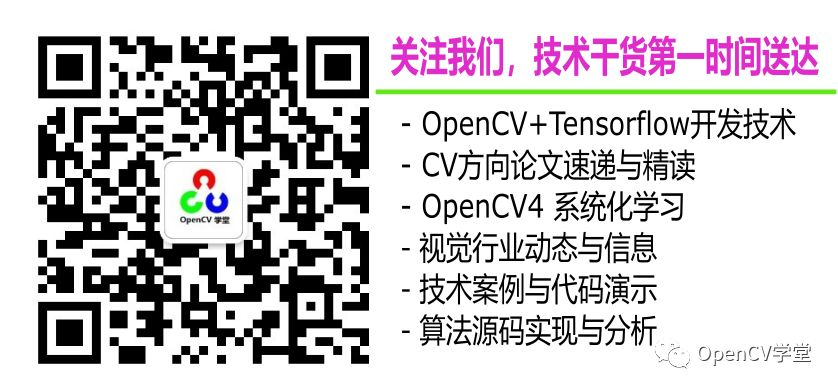展开全文• ## 有关于图像频率域和滤波的总结

万次阅读 多人点赞 2015-12-01 14:45:11
前段时间看了很多的概念和知识，发现因为是走马观花的过了一遍，所以看得稀里糊涂的，然后许多地方混淆了概念，特别是关于图像频率域的部分的理解（包括图像频率域滤波之类的），所以下面总结一下这段时间重新看...

前段时间看了很多的概念和知识，发现因为是走马观花的过了一遍，所以看得稀里糊涂的，然后许多地方混淆了概念，特别是关于图像频率域的部分的理解（包括图像频率域滤波之类的），所以下面总结一下这段时间重新看《数字图像处理》（电子工业出版社，Matlab本科教学版）第三章重新收获的关于频率域的理解。
那么什么是图像的频率域呢？理解了图像的频率的概念，就不难理解频率域。我个人理解是这么类比的，图像可以看成是一个特殊的二维的信号，然后某一点的灰度级，其实就是图像信号上这一点的”幅度“，那么根据信号的概念，频率就是信号变化的快慢，这样就好理解了，所谓的频率也就是这个图空间上的灰度变换的快慢，或者是叫图像的梯度变化，什么地方梯度频率比较大呢？这在图像中自然是“边界”比较大。举个例子来讲，如果一幅图整体变化不大（比如说是一面墙的图），那么他在频率域下低频成分就很多，而高频成分就极少。而显然如果是一幅国际象棋棋盘，他的高频成分相对刚才那幅墙的图片来说，肯定多得多。
然后从图像域变换到频率域，我们用的函数就是大名鼎鼎的二维离散傅里叶变换了:
令f(x,y)表示一幅大小为MXN像素的数字图像，其中，x=0，1，2······M-1, y=0，1，2······N-1，由F(u，v)表示的f(x，y)的二维离散傅里叶变换（DFT）由下式给出：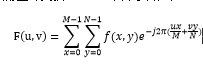式子当中，u也是属于0到M-1,v属于0到N-1。频率域就是属于u，v作为频率变量，由F(u，v)构成的坐标系，这块MXN的区域我们通常称为频率矩形，很明显频率矩形的大小和输入图像的大小相同。
有傅里叶变换，当然就有傅里叶反变换(IDFT)：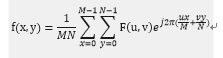这里多提一句，按照书上的说法，在DFT的表达式中，1/MN项出现在正变换前面，而在有些表达式中就如上式那样出现在反变换前面，因为Matlab采用的是后者，所以书上的公式就是用的上式那种了。
由上面的公式可以看出几个点：
1.    不难看出，F(0，0)等于f(x,y)平均值的MN倍,因为带入到傅里叶变换公式中可以得到F(0，0)实际上就是图像中所有f(x，y)求和。所以我们也常把F(0，0)称作傅里叶变换的直流分量。
2.    我们可以很明显的看出，在频率域中的一点(u,v)的值F(u，v)，他并不决定于空间域中（x,y）的值，而是跟整个图像空间域中的所有的点都有关系，因此，在频率域中的一点(u,v)的值，例如说F(5,5)和空间域上的这一点f(5,5)并没有什么关系，而是决定于整幅图像。
接下来书上讲了傅里叶变换的一些性质，还有傅里叶谱，变换的相角定义，功率谱定义等等概念，因为打公式太麻烦，这里就不多赘述了，可以在网上找到现成的公式定义和概念。
傅里叶变换性质里面个人认为比较重要的是周期性，因为周期性的缘故，所以在傅里叶变换过后的频率图里，四角的是低频分量（具体的我也不太清楚，但是比如低频分量），我们到时候会把低频分量移到中心位置来，因为大部分图像的低频分量其实更多，所以会在边角上比较亮，放到中间来比较好看。
下面举例一幅图说明看一幅频率域傅里叶谱图:
f=imread('3.jpg');
imshow(f);%显示原图
F=fft2(f);%做傅里叶变换
S=abs(F);%取傅里叶谱

figure,imshow(S,[]);%显示傅里叶谱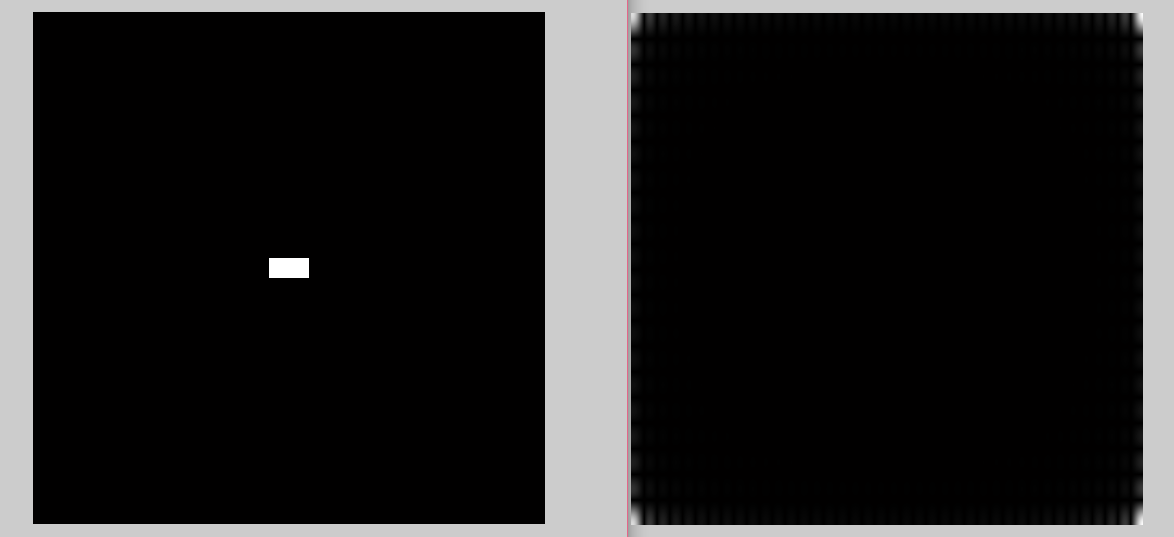左图是原图，右图是频率图，频率图中的亮暗说明了对应频率的多少，上面已经谈过，如果从右图可以看出来是边上的四个点比较亮，这说明原图中低频分量比较多，也就是说原图中的变化比较少。换句话来说，如果原图看成地形的话，那说明大部分地形非常平坦。
我们可以通过公式（欧拉公式e^(2*pi*j)=1以及指数的计算），可以很容易发现（图是盗的- -）：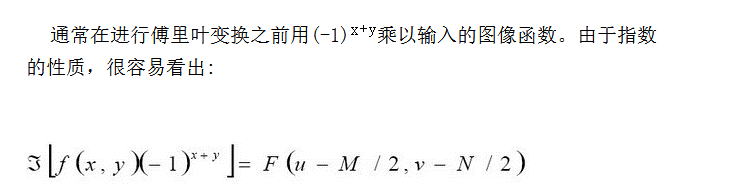上式最左边那个符号表示求傅里叶变换。由上式知道这么弄一番之后，是可以把频率图的原点放到中心的。
在matlab中用的是fftshift函数。
居中过后的频率谱显示如下：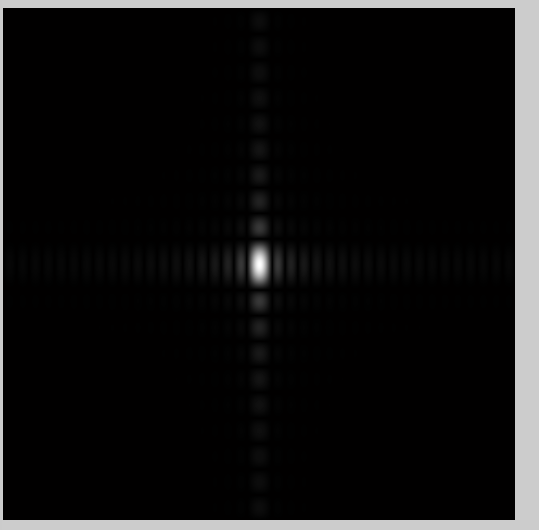可以看得更清楚一些，这个时候因为其实频率谱的范围是很大的，相对于低频分量来说，高频分量的值比较少，所以可以用对数变换(log(1+abs(F))将高频细节扩展出来，显示如下：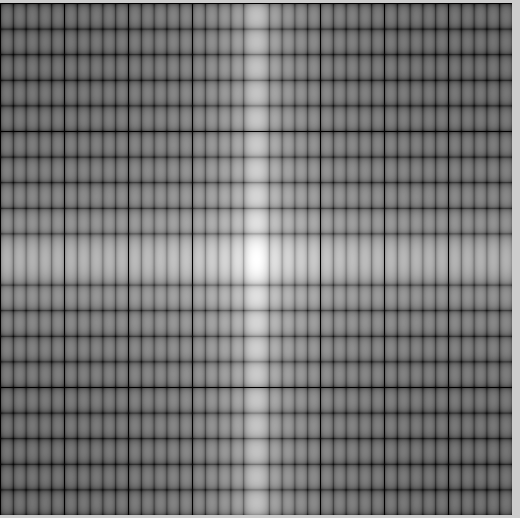细节还是突出的比较明显的哈！
下面谈谈对滤波的理解，不知道是不是完全正确。
滤波和算子都是数字图像处理的基本操作，其中滤波是指在像素领域（空间域）内做领域处理(中值滤波、均值滤波)或者在图像频域内（需要先做傅里叶变换）做处理（低通滤波、高通滤波、带通滤波）。算子一般就是指的是卷积算子了。
先来谈谈空间域的滤波。什么叫领域处理呢？领域处理的就是对领域进行一系列的操作：
(1)    选取中心点(x,y)；
(2)    仅对预先定义的点（x,y）的领域内的像素执行操作;
(3)    令运算结果为该点处的响应；
(4)    对图像中的每一点重复该处理。
中心点移动的过程中会产生新的领域，每个领域对应输入图像上的一个像素。用来标识该处理的两个主要术语是领域处理和空间滤波，其中后者更为通用。如果对领域中像素执行的计算是线性的，则称该操作是线性空间滤波。（也用术语空间卷积）；否则称为非线性空间滤波。
线性操作包括领域中的每个像素乘以相应的系数，将结果求和，从而得到点(x,y)处的响应。若领域的大小为mxn，则需要mn个系数。这些系数被排列为一个矩阵，称为滤波器(模板)/滤波模板/核/掩模或者窗口。其中前三个属于最常见。为变得更明显一些，也用卷积滤波、卷积模板或者卷积核等术语。
再来说下卷积算子是个什么意思：
在这里盗一下网上的图：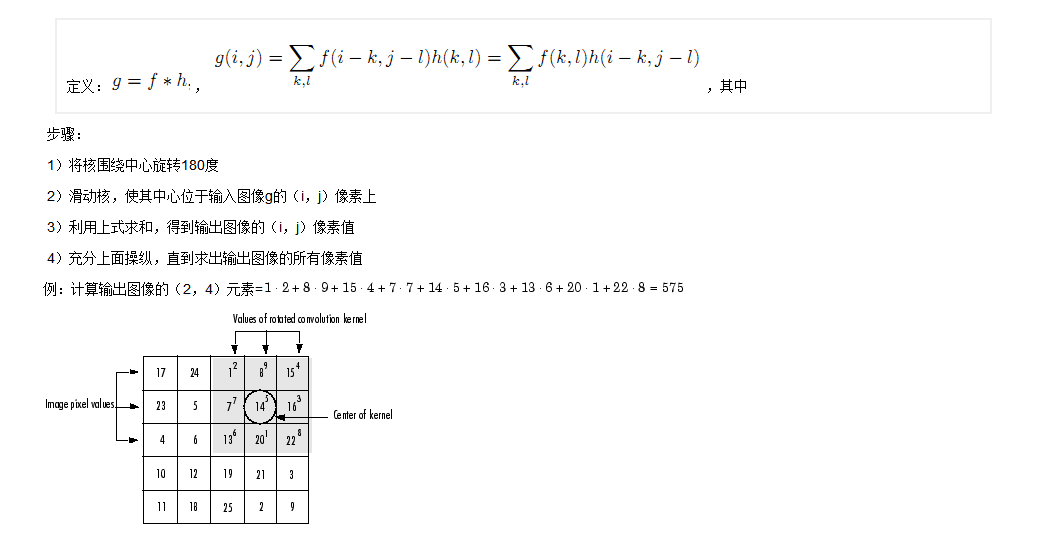然后如上所示，图片中右上角的那个矩阵h=[2 9 4;
7 5 3;
6 1 8;]
就是后面经常提到的模板了，在相关书本上一般也写作w(x,y)。如果把基于乘积和的值用来替代成原来的(x,y)的值，那么这种滤波方式叫做线性滤波（比如拉普拉斯算子）。其他的叫做非线性滤波。
空间域滤波的最常见的就是中值滤波和均值滤波，顾名思义，中值滤波就是用图像领域中所包含的像素的排序集合的中值进行替代，而均值滤波是用领域像素们的均值进行滤波。中值滤波也属于统计排序滤波。
接下来介绍频率域的滤波：
首先，有个定理，叫做卷积定理：
f(x,y)★h(x,y)óH(u,v)F(u,v)
上式中★代表卷积，ó代表一个傅里叶变换对。
频率域的滤波比较多的就是高斯低通/高斯高通滤波器了，和空间域稍微有区别的是，在频率域内不是进行的领域处理，而是进行的是函数修改：
G(u，v)= H(u,v)F(u,v)
函数H(u,v)又叫做滤波器传递函数，频率域滤波的思想就是选择一个滤波器传递函数，该函数按照指定的方式修改F(u，v)。
由卷积定理可知，在频率域中让F(u,v)乘以空间滤波器的傅里叶变换H(u，v)，再做一个傅里叶反变换，就可以得到用空间域滤波器滤波后的图像了（这也是相当于用DFT的方式在做卷积，用这种方式做卷积又叫循环卷积）。
值得一提的是，注意，当作循环卷积的时候，因为F和H都是周期函数，所以G必然也是周期函数，在周期接近函数非零部分的持续周期时对周期函数进行卷积会引起相邻周期的串扰(这种串扰又叫折叠误差)，所以要通过补零的方法来避免。比较拗口，还是举例来说明：一个上黑下白的方块图片，在DFT卷积的时候我们假设在处理上边沿了，结果因为是周期的所以上边沿其实在处理时会受到上边沿上面另一个方块的下边沿的白色部分的影响（具体的例子见书P63页），补0之后就不会受影响了。


展开全文二维离散傅里叶变换
• https://zhuanlan.zhihu.com/p/19763358?columnSlug=wille https://blog.csdn.net/u011178262/article/details/84674412 https://blog.csdn.net/forrest02/article/details/55510711
https://zhuanlan.zhihu.com/p/19763358?columnSlug=wille

https://blog.csdn.net/u011178262/article/details/84674412

https://blog.csdn.net/forrest02/article/details/55510711

展开全文• 文章目录傅里叶变换基础傅里叶级数傅里叶积分傅里叶变换一维连续傅里叶变换一维离散傅里叶变换二维离散傅里叶变换正变换反变换卷积卷积定理数字图像DFT空间和频域图像频域滤波基本步骤图像频率特性分析图像滤波...
文章目录傅里叶变换基础傅里叶级数傅里叶积分傅里叶变换一维连续傅里叶变换一维离散傅里叶变换二维离散傅里叶变换正变换反变换卷积卷积定理数字图像DFT空间域和频域图像频域滤波基本步骤图像频率特性分析图像滤波实践Python分析C++分析源代码参考资料
傅里叶变换基础
傅里叶级数
法国数学家傅里叶发现，任何周期函数都可以用正弦函数和余弦函数构成的无穷级数来表示（选择正弦函数与余弦函数作为基函数是因为它们是正交的），即 任何周期信号都可以表示成一系列正弦信号的叠加

三角形式

$f(t) = \frac{a_0}{2} + \sum_{k=1}^{+\infty} \big[ a_k cos (n \omega t) + b_k sin (n \omega t) \big], \quad \frac{a_0}{2} = \frac{1}{T} \int_{-\frac{T}{2}}^{\frac{T}{2}} f(t) dt$

复指数形式

$f(t) = \frac{1}{T} \sum_{n=-\infty}^{+\infty} [ \int_{-\frac{T}{2}}^{\frac{T}{2}} f(\tau)e^{-j\omega_n\tau} d\tau ] e^{j\omega_nt}$
基波角频率 $\omega = \frac{2\pi}{T}$ ， $T$ 为 $f(t)$ 的周期， $j$ 为虚数单位
傅里叶积分
复指数形式
$f(t) = \frac{1}{2\pi} \int_{-\infty}^{+\infty} [ \int_{-\infty}^{+\infty} f(\tau)e^{-j\omega\tau} d\tau ] e^{j\omega t} d\omega$
傅里叶变换
一维连续傅里叶变换
正变换 为
$F(\omega) = \int_{-\infty}^{+\infty} f(t) e^{-j\omega t} dt$
逆变换 为
$f(t) = \frac{1}{2\pi} \int_{-\infty}^{+\infty} F(\omega) e^{j\omega t} d\omega$
一维离散傅里叶变换
正变换 为
$F(u) = \sum_{x=0}^{M-1} f(x) e^{-j2\pi \frac{ux}{M}}$
则
$F(0) = \sum_{x=0}^{M-1} f(x)$
反变换 为
$f(x) = \frac{1}{M} \sum_{u=0}^{M-1} F(u) e^{j2\pi \frac{ux}{M}}$
对于反变换式前的系数 $\frac{1}{M}$ ，也可放在正变换中，只要保证正变换与反变换之前的系数乘积为 $\frac{1}{M}$ 即可。
二维离散傅里叶变换
正变换
二维离散傅里叶变换：
$F[f(x,y)] = F(u,v) = \sum_{x=0}^{M-1} \sum_{y=0}^{N-1} f(x,y) e^{-j2\pi(\frac{ux}{M}+\frac{vy}{N})}$
当 $(u,v)$ 等于 $(0,0)$ 时，直流分量 为：
$F(0,0) = \sum_{x=0}^{M-1} \sum_{y=0}^{N-1} f(x,y)$
幅度谱 为：
$A(u,v) = |F(u,v)| = \sqrt{Real(u,v)^2 + Image(u,u)^2}$
功率谱 为：
$P(u,v) = |F(u,v)|^{2} = Real(u,v)^2 + Image(u,u)^2$
相位谱 为：
$\phi(u,v) = arctan \frac{Image(u,v)}{Real(u,v)}$
通过 幅度谱 和 相位谱，我们也能合成 其傅里叶变换（频谱）：
\begin{aligned} F(u,v) &= A(u,v)e^{j\phi(u,v)} \\ &= A( cos \phi + jsin \phi ) \quad \text{(省略(u,v)，应用 欧拉公式)}\\ &= Acos\phi + jAsin\phi \end{aligned}
注意：

上面式子中的 $j$ 为 虚数单位
$Real(u,v)$  为 复数的 实部
$Image(u,v)$ 为 复数的 虚部

反变换
$f(x,y) = F^{-1}(u,v) = \frac{1}{MN} \sum_{u=0}^{M-1} \sum_{v=0}^{N-1} F(u,v) e^{j2\pi(\frac{ux}{M}+\frac{vy}{N})}$
卷积
$\int_{-\infty}^{+\infty} f_1(\tau)f_2(t-\tau) d\tau = f_1(t) * f_2(t)$
卷积定理
函数卷积的傅立叶变换是函数傅立叶变换的乘积

时域卷积定理：时域内的卷积对应频域内的乘积

$F[f_1(t) * f_2(t)] = F_1(\omega) \cdot F_2(\omega)$

频域卷积定理：频域内的卷积对应时域内的乘积

$F[f_1(t) \cdot f_2(t)] = \frac{1}{2\pi} F_1(\omega) * F_2(\omega)$
数字图像DFT
借用知乎大神Heinrich的一张图，来个感性认识：空间域和频域

空间域：在图像处理中，时域可以理解为 空间域 或者 图像空间，处理对象为图像像元；
频域：以 空间频率 为自变量描述图像的特征,可以将一幅图像像元值在空间上的变化分解为具有不同振幅、空间频率和相位的简振函数的线性叠加，图像中各种空间频率成分的组成和分布称为 图像频谱

空间域与频域可互相转换，对图像施行 二维离散傅立叶变换 或 小波变换 ，可以将图像由空间域转换到频域；通过 对应的反变换 又可转换回空间域图像，即人可以直接识别的图像。
图像频域滤波
二维数字图像的滤波主要分为 空间域滤波 和 频域滤波：

空间域滤波： 用各种模板直接与图像进行 卷积运算，实现对图像的处理，这种方法直接对图像空间操作，操作简单

频域滤波： 在实现某些图像处理的时候，频域的处理比空间域更简单；对于在空间域上的数字图像，根据 卷积定理 可以通过 傅立叶变换 将 空域卷积滤波 变换为 频域滤波，然后再将频域滤波处理后的图像 反变换 回空间域

基本步骤
图像频域滤波步骤为（频谱图中心化）：

计算 原始图像 $f(x,y)$ 的DFT，得到 频谱 $F(u,v)$
中心化：将频谱 $F(u,v)$ 的零频点移动到频谱图的中心位置
计算 滤波器函数 $H(u,v)$ 与 $F(u,v)$ 的乘积 $G(u,v) = F(u,v) \cdot H(u,v)$
反中心化：将频谱 $G(u,v)$ 的零频点移回到频谱图的左上角位置
计算上一步计算结果的 傅里叶反变换 $g(x,y)$
取 $g(x,y)$ 的 实部 作为最终滤波后的结果图像

上面步骤是对 图像频谱 进行 中心变换；我们也可以先对 原始图像 进行 中心变换，再计算其 频谱图，滤波步骤如下（原始图中心化）：

原始图像 $f(x,y)$ 中心变换：$f(x,y) \cdot (-1)^{(x+y)}$
计算上一步计算结果的DFT，得到其 频谱 $F(u,v)$
计算 滤波器函数 $H(u,v)$ 与 $F(u,v)$ 的乘积 $G(u,v) = F(u,v) \cdot H(u,v)$
计算 $G(u,v)$ 的 傅里叶反变换 $g(x,y)$
取 $g(x,y)$ 的 实部
上一步计算结果 乘以 $(-1)^{(x+y)}$ 作为最终滤波后的结果图像

滤波能否取得理想结果的关键取决于上面的 滤波器函数 $H(u,v)$ 。
这时让我想到了《自动控制理论》中的 传递函数 $G(s)$，定义为：初始条件为零的线性定常系统输出的拉普拉斯变换与输入的拉普拉斯变换之比。
下面以 控制论的思想 给出图像频域滤波的示意框图：图像频率特性分析
频谱图上的每一个像素点都代表一个频率值，幅值由像素点亮度变码而得。对于一幅图像，图像信号的 频率特性 如下：

直流分量 表示预想的平均灰度
低频分量 代表了大面积背景区域和缓慢变化部分
高频分量 代表了它的边缘、细节、跳跃部分以及颗粒噪声
振幅 描述了图像灰度的亮度
相位 决定了图像是什么样子

数字图像的二维离散傅立叶变换所得的结果的频域成分如下图所示，左上角是直流成分，变换结果四个角周围对应于低频成分，中央部分对应于高频部分为了便于观察，常常采取 换位 方法使直流成分出现在窗口的中央（中心化），变换后中心为低频，向外是高频。
在频域，可以很方便的实现 图像的锐化和模糊：

截取频率的低频分量，对其作傅立叶反变换，得到的就是模糊后的图像，即 低通滤波
截取频率的高频分量，对其作傅立叶反变换，得到的就是锐化后的图像，即 高通滤波

图像滤波实践
下面，我们以 lena.bmp(点此下载) 图像进行滤波实践。
Python分析
（1）加载图像，并转换为 灰度图 为（2）对其 快速傅里叶变换，并经过 中心变换，得到 频率谱 和 相位谱（3）分别截取 频谱图 的 低频部分（中间部分） 和 高频分量（四周部分）（4）对以上处理过的频谱图分别进行 反中心化、傅里叶反变换、取实部，得到 低通滤波 和 高通滤波 后的图像C++分析
使用 CImg 和 FFTW库 对 lena图像进行傅里叶变换（源代码见文末），结果如下源代码
以上所有代码均存储在我的Github仓库：

Python Code: cggos/cgocv_app/cv_py
C++ Code: cggos/cgocv_app/image_process/fftw_demos

参考资料

傅里叶分析之掐死教程
Fourier Transforms (scipy.fftpack)
Python下opencv使用笔记（十）（图像频域滤波与傅里叶变换）
Fourier Transform (OpenCV-Python Tutorials)
SIGNAL PROCESSING WITH NUMPY II - IMAGE FOURIER TRANSFORM : FFT & DFT
《数字图像处理与计算机视觉——Visual C++与MATLAB实现》
《积分变换》


展开全文• 我们最熟的应该是时域特征（图像里面叫空间比较好）啦，我的理解是因为这是我们所直接看到的或者所直接感觉到的。 既然图像可以看做信号的话，我们当然可以把图像也变换到频域上去分析，连接时域与频域的通道便...
• 这是能够实现图像频率域增强的C++代码 在频率域中实现增强图像的效果
• 3. Butterworth和Gauss图像频率域低通滤波； 4. Butterworth和Gauss图像频率域高通滤波； 3. 巴特沃斯和高斯图像频率域低通滤波； 4. 巴特沃斯和高斯图像频率域高通滤波； 内含文档、Matlab代码、结果图
• matlab频率域图像处理代码和实验报告-实验、频率域图像处理.rar 频率域图像处理 实验三、频率域图像处理 （一）实验目的： 1、理解图像的傅立叶变换； 2、掌握频率域滤波的基本方法。 （二）实验要求： 1...matlab
• 文章目录图像频率域增强频率域低通滤波器H(u,v)理想低通滤波器Butterworth低通滤波器指数低通滤波器梯形低通滤波器 图像频率域增强 频率域低通滤波器H(u,v) 理想低通滤波器 由于高频成分包含有大量的边缘信息，...
• 主要的话题：图像傅里叶变换理论...傅里叶变换的基本性质变换，变换，频率域运算。 连续傅里叶变换及其性质，取样标准，离散傅里叶变换和性质，图像变换，变换的对偶。逆傅里叶变换。 旋转和缩放。 原理叠加和线性度。
• matlab开发-图像恢复频率域WienerFilter。频域图像恢复（维纳滤波器）
• 频率域基础请参考“图像处理之频率域数学基础”。 通过傅里叶变换，可以把图像从空间域转换到频率域图像的频率是表征图像中灰度变化剧烈程度的指标，是灰度在平面空间上的梯度。频率直接关系到图像空间灰度的变化...高斯滤波器 低通滤波器 带阻滤波器
• 图像频率域:是图像像元的灰度值随位置变化的空间频率，以频谱表示信息分布特征，傅立叶变换能把图像从空间域变换到只包含不同频率信息的频率域，原图像上的灰度突变部位、图像结构复杂的区域、图像细节及干扰噪声...image Image
• 内容简介频率域去噪带阻滤波器带通滤波器陷波滤波器最佳陷波滤波器 频率域去噪 为了节约读者时间，首先说明这一篇不会有任何代码只有个人的心得体会理由如下： 代码过于简单，像书上这样的例子这样的 自己并没有这类...
• 1、在图像中，像元的灰度值随位置变化的频繁程度可以用频率来表示，这是一种随位置变化的空间频率。 ...是指连续像元的灰度值的最高值与最低值的差。... 滤波是指在图像空间域(x,y)或者频率域...
• 通过离散余弦变换，离散傅里叶变换和小波变换说明了二维图像转换为频率域的情况
• 【项目程序】对图像进行频率域图像增强，计算并画出此图像的中心化频率谱，用拉普拉斯算子对此图像锐化.rar
• 转自：http://blog.csdn.net/forrest02/article/details/55510711频率域在介绍频率域图像处理之前，先提几个问题。 1.什么是频率域？ 2.为什么要在频率域中进行图像处理？频率域的概念 频率域是指从函数的频率...
• 频率域图像增强：以修改图像的傅里叶变换为基础的方法； 高、低通滤波，同态滤波。 另外还有彩色图像的增强。 1.空间域图像增强 1.1基于灰度变换的图像增强 属于点处理技术，这部分内容参考之前的笔记点运算部分...
• 图像增强处理：设计一套空间域与频率域结合的图像增强算法，处理以下任一组图片中的带噪声图像，去除噪声，提高图像质量。 （1）已知：噪声为随机噪声和周期噪声混合噪声； （2）要求： a）去噪处理后，计算均方误差...
• 目录频率域滤波基础频率域的其他特性频率域滤波基础知识频率域滤波步骤小结空间域和频率域滤波之间的对应关系 频率域滤波基础 频率域的其他特性 频率域中的滤波过程如下： 首先修改傅里叶变换以在到特定目的 然后...深度学习 python numpy...